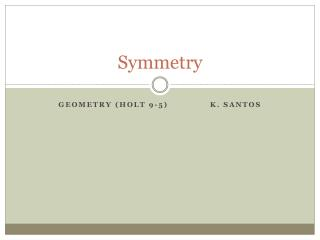DownloadDownload PresentationSymmetry

# Symmetry

Télécharger la présentation## Symmetry

- - - - - - - - - - - - - - - - - - - - - - - - - - - E N D - - - - - - - - - - - - - - - - - - - - - - - - - - -
##### Presentation Transcript

1. Symmetry Geometry (Holt 9-5) K. Santos

2. Symmetry A figure has symmetry if there is a transformation (change) of the figure such that the image coincides with the preimage (original image) Can you fold it onto itself exactly? Can you rotate it without anyone knowing? There are two types of symmetry: Reflectional Symmetry----folding Rotational Symmetry------spinning/rotating

3. ReflectionalSymmetry (line symmetry) A figure has reflectional symmetry (line symmetry) if it can be reflected across a line so that the image coincides with the preimage. Fold the figure along the line of symmetry and the halves match up exactly ReflectionalSymmetry—one half of the image is a mirror image of its other half The line of symmetry (axis of symmetry) divides the figure into two congruent halves.

4. Examples of Figures with Reflectional Symmetry

5. Examples of Letters with Reflectional Symmetry HELLO MATH STUDENTS! Letters with reflectional (line) symmetry: H, E, O, M, A, T, U, D HELLO MATH STUDENTS! Letters with reflectional (line) symmetry: H, E, O, T, D

6. Rotational Symmetry (Radial Symmetry) A figure that has rotational symmetryif it can be rotated about a point by an angle greater than 0 and less than 360 so that the image coincides with the preimage. Can rotate or spin a figure around and can’t even tell the figure has been moved Full circle is 360 -- if this is the only rotation possible then the figure does not have rotational symmetry The angle of rotational symmetry is the smallest angle through which a figure can be rotated to coincide with itself The number of times the figure coincides with itself as it rotates 360 is called the order of the rotational symmetry

7. Examples of Figures with Rotational Symmetry (360) ¼(360) 180 every 90 order: 2order: 4 1/3(360) 1/5 (360) every 120 every 72 order: 3 order: 5

8. Examples of Figures with Rotational Symmetry ½(360) ¼(360) 1/12(360) 180 every 90 every 30 Order: 2 order: 4 order: 12 has rotational symmetry for any degree from 0 to 360

9. Point Symmetry Has rotational symmetry of exactly 180 Can turn a figure upside down